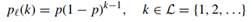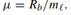Create an Account

Home / Questions / A link with transmission rate Rb is used to forward packets having random size which is as...

A link with transmission rate Rb is used to forward packets having random size which is assumed to have geometric PMD Prove tha if m 1/p is large enough the distribution of the packet

1. A link with transmission rate Rb is used to forward packets having random size , which is assumed to have geometric PMDProve that, if m = 1/p is large enough, the distribution of the packet transmission times can be approximated to an exponential distribution with parameter2. A dentist clinic opens up for business at t = 0. Customers arrive according to a Poisson rp with rate λ. Each medical treatment takes a random time y. Find the probability P that the second arriving customer will not have to wait and the average value mw of his waiting time for the two following cases:

a) y = c =constant.

b) y is exponentially distributed with parameter µ = 1/c.

Jul 31 2020 View more View LessSubscribe To Get Solution